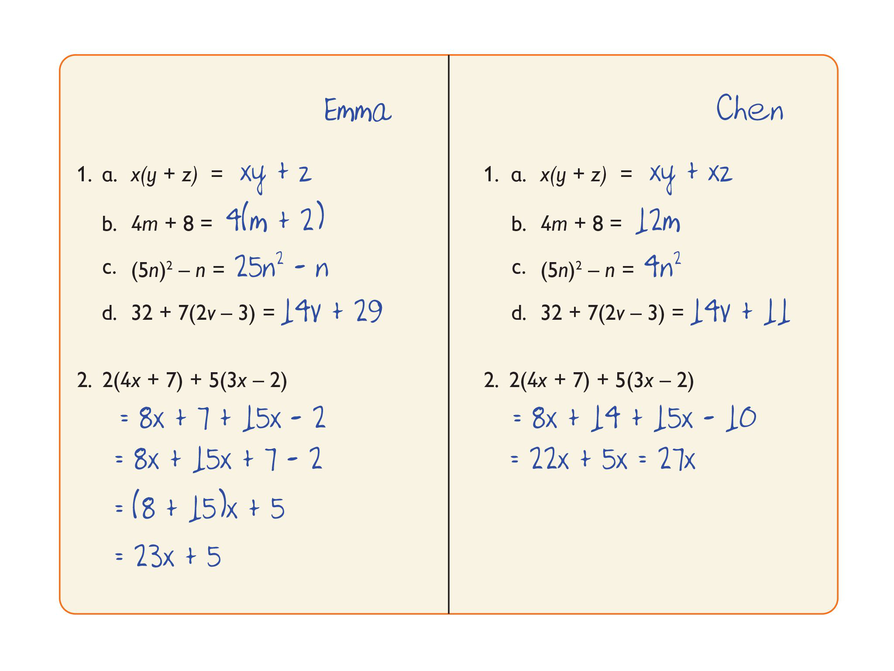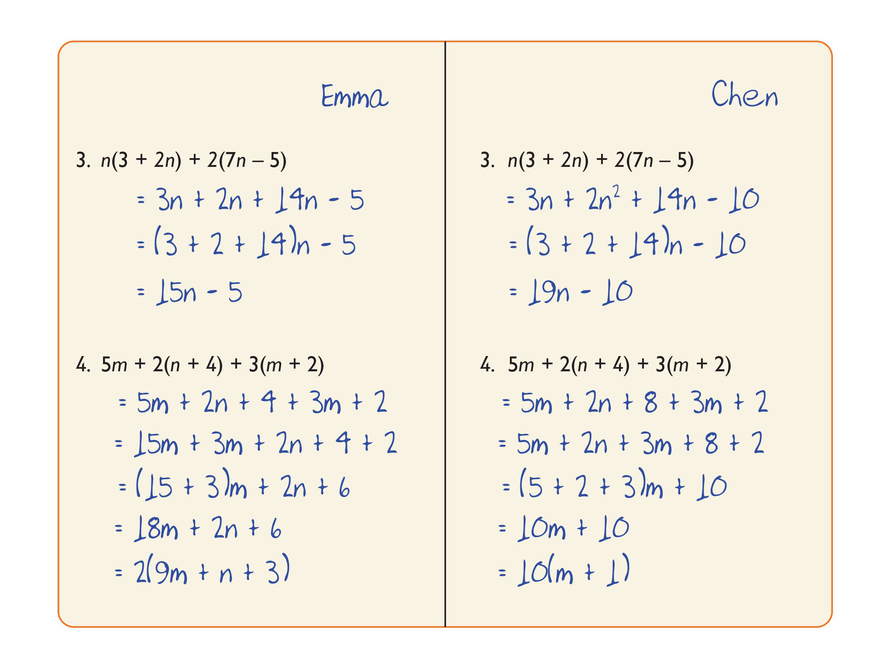Subject:
Mathematics
Material Type:
Lesson Plan
Level:
Middle School
6
Provider:
Pearson
Tags:
Language:
English
Media Formats:
Text/HTML

# Peer Review## Overview

Students critique the work of other students and revise their own work based on feedback from the teacher and peers.

# Key Concepts

Concepts from previous lessons are integrated into this unit task: rewriting expressions, using parentheses, and using the distributive property. Students apply their knowledge, review their work, and make revisions based on feedback from you and their peers. This process creates a deeper understanding of the concepts.

# Goals and Learning Objectives

• Apply knowledge of expressions to correct the work of other students.
• Track and review the choice of strategy when problem solving.

# Lesson Guide

Return students’ solutions to the Self Check task from Lesson 10. If you have not added questions to individual pieces of work, write your list of questions on the board now. Students can then select questions appropriate to their own work.

Have students read over the feedback they received and give them a few minutes to think about it.

# Critique

Revise your work on the Self Check problem from the last lesson based on the following questions.

• How can you tell if Emma's and Chen's work (from the previous Self Check problem) is correct or incorrect?
• Can you find one problem that you know was done incorrectly?
• Can you find one problem in which the distributive property was used incorrectly?
• What do parentheses in a problem tell you?
• Can you explain why the work you identified as incorrect is not right?

# Lesson Guide

Discuss the Math Mission. Students will use the distributive property and work with expressions to find and fix student errors.

## Opening

Use the distributive property and work with expressions to find student errors and fix them.

# Lesson Guide

Support student problem solving.

• Try not to make suggestions that move students toward a particular approach to this task. Instead, ask questions that help students clarify their thinking.
• If students find it difficult to get started, these questions might be useful:
• What feedback questions were you asked?
• How could you and your partner work together to address one of those feedback questions?

If several students in the class are struggling with the same issue, you could write a relevant question on the board. You might also ask a student who has performed well on a particular part of the task to help a struggling student.

SWD: For some students you will be able to provide questions that can prompt a student’s reflection. However, if you have some students who have been completely unsuccessful on the Self Check, marking their paper may be counterproductive. Meet with them in a small group and rework the problem as a group to deepen students’ understanding.

# Mathematical Practices

Mathematical Practice 1: Make sense of problems and persevere in solving them.

Identify students who make sense of problems and persevere in solving them as they review and revise their work based on feedback.

Mathematical Practice 3: Construct viable arguments and critique the reasoning of others.

Look for students who successfully critique the reasoning of others, both in the task itself and when evaluating the work of peers.

# Interventions

Student has difficulty getting started.

• What feedback did you get?
• How can you use the feedback to revise your work?

Student works unsystematically.

• How can you check that you addressed all the feedback?

Student presents his work poorly.

• Have you given enough explanation?

Student has a correct solution.

• What method did you use to identify and correct the student work that is wrong?

1. Problem 1

• Emma does not distribute the x over both the y and z. Chen answers correctly.
• Emma answers correctly. Chen incorrectly adds the unlike terms 4m and 8.
• Emma answers correctly. Chen incorrectly subtracts the unlike terms (5n)2 − n.
• Emma does not distribute the 7 correctly. She subtracts 3 from 32 instead. Chen answers correctly.

Problem 2

• Emma again does not distribute the number in front of the parentheses correctly. She does not multiply the second term in the parentheses.
• Chen incorrectly adds the terms 8x and 14 to get 22x, and subtracts 10 from 15x to get 5x. The correct answer is 23x + 4.
2. The most common type of error that Emma made was that she did not distribute a multiplier over a whole quantity in parentheses.

3. The most common type of error that Chen made was that he added terms that are not alike.

# Correct Student Work

Work with a partner and try the Self Check task again—this time combining your ideas.

1. Imagine that you are the teacher. Decide whether the work shown is correct or incorrect. If you see an incorrect answer, do the following:

• Cross it out and replace it with a correct answer.
• Explain what is wrong using words or diagrams.
2. What was the most common type of error that Emma made?

3. What was the most common type of error that Chen made?# Lesson Guide

Support student problem solving.

• Try not to make suggestions that move students towards a particular approach to this task. Instead, ask questions that help students to clarify their thinking.
• If students find it difficult to get started, these questions might be useful:
• What feedback questions were you asked?
• How could you and your partner work together to address one of those feedback questions?

If several students in the class are struggling with the same issue, you could write a relevant question on the board. You might also ask a student who has performed well on a particular part of the task to help a struggling student.

SWD: Demonstrate and verbally explain to your students what needs to be done, and rephrase the instructions as necessary. This problem incorporates a hands-on activity that integrates vocabulary and mathematical concepts, as well as reading, listening, and speaking.

# Mathematical Practices

Mathematical Practice 1: Make sense of problems and persevere in solving them.

Identify students who make sense of problems and persevere in solving them as they review and revise their work based on feedback.

Mathematical Practice 3: Construct viable arguments and critique the reasoning of others.

Look for students who successfully critique the reasoning of others, both in the task itself and when evaluating the work of peers.

# Interventions

Student has difficulty getting started.

• What feedback did you get?
• How can you use the feedback to revise your work?

Student works unsystematically.

• How can you check that you addressed all the feedback?

Student presents his work poorly.

• Have you given enough explanation?

Student has a correct solution.

• What method did you use to identify and correct the student work that is wrong?

1. Problem 3

• Emma does not distribute the multipliers n and 2 correctly. She does not multiply the second term. She also adds 3 + 2 + 14 incorrectly and gets 15 rather than 19.
• Chen again combines unlike terms. He factors out n from 2n2 to get 2. The correct answer should be 2n2 + 17n − 10.

Problem 4

• Emma does not distribute the multipliers 2 and 3 correctly. She does not multiply the second terms by 2 and by 3. She also mis-copies 5m as 15m.
• Chen again incorrectly combines unlike terms. He factors out m from 5m + 2n + 3m to get 10m. He also makes the same mistake as Emma (but only once) and forgets to multiply 3 and 2 in 3(m + 2). The correct answer should be 8m + 2n + 14.

2. The most common type of error that Emma made was that she did not distribute a multiplier over a whole quantity in parentheses.

3. The most common type of error that Chen made was that he added terms that are not alike.

# Emma's and Chen's Work

1. Emma and Chen have done more work. With your partner, decide whether their work is correct or incorrect. If you see an incorrect answer, do the following:

• Cross it out and replace it with a correct answer.
• Explain what is wrong using words or diagrams.
2. What was the most common type of error that Emma made?

3. What was the most common type of error that Chen made?# Preparing for Ways of Thinking

While students work in small groups with their partners, note different student approaches to the task.

• How do students organize their work?
• Do they notice if they have chosen a strategy that does not seem to be productive? If so, what do they do?

Select some different approaches for the Ways of Thinking.

# Challenge Problem

• 2+ 13
• x3 + y2
• 2x + 4y
• Explanations will vary.

# Prepare a Presentation

Explain how you would work with Emma and Chen to improve their work.

# Challenge Problem

Write an expression that:

• Is a sum of two terms using a variable of your choice.
• Has exponents 3 and 2
• One coefficient is 2 and the other is 4.
• Compare your expression with that of your partner. Can you make a different expression? Explain why or why not?

# Mathematics

Organize a whole class discussion to consider issues arising from students’ revision work. You may not have time to address all these issues, so focus your class’s discussion on the issues that are most important for your students.

Have students share their work and discuss how they approached correcting the student work.

Have students whose strategies did not work share, so they can talk about how and when they realized their strategy did not work and what they did about it.

Have students share the questions from you or the computer that they addressed and how they addressed those questions.

Have students ask questions and make observations as they view each other’s work.

# Ways of Thinking: Make Connections

## Hint:

• What strategy did you use to find the student errors?
• How could you tell if the work was correct or incorrect?
• How does your explanation or diagram clearly show what the student did wrong?
• How did what you have learned about expressions and the distributive property help you evaluate the student work?
• How did you identify the most common error that each student made?
• What advice would you give each student about how to deal with problems of this type in the future?